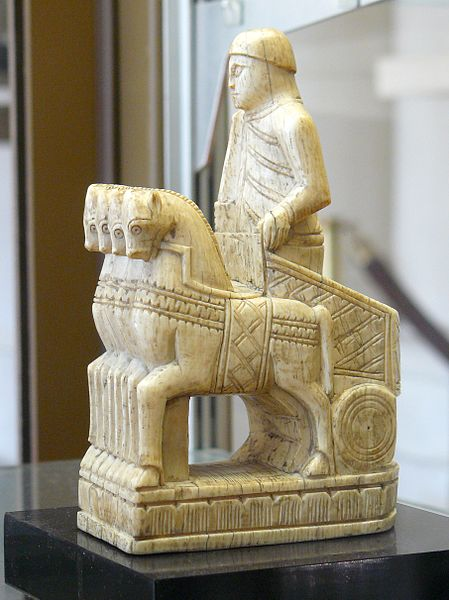# Minimum Number Of Rooks

Probability Level 5Find the smallest positive integer $k$ for which the following statement is true.

Suppose $k$ rooks are placed on a $2014 \times 2014$ chessboard. Then, there must exist three rooks, call them $r_1, r_2, r_3,$ such that $r_1$ attacks $r_2,$ $r_3$ also attacks $r_2$, but $r_1$ doesn't attack $r_3$.

Details and assumptions

• Two rooks are said to attack each other if they lie on the same row or same column.
• The $k$ rooks are placed on distinct cells, i.e. a cell contains at most one rook.
• The given condition must hold for any configuration of $k$ rooks.
• This problem is not original.
###### Image credit: Wikipedia Siren-Com
×

Problem Loading...

Note Loading...

Set Loading...